Test: Casting Level - 3

# Test: Casting Level - 3

Test Description

## 20 Questions MCQ Test Manufacturing Engineering | Test: Casting Level - 3

Test: Casting Level - 3 for Mechanical Engineering 2023 is part of Manufacturing Engineering preparation. The Test: Casting Level - 3 questions and answers have been prepared according to the Mechanical Engineering exam syllabus.The Test: Casting Level - 3 MCQs are made for Mechanical Engineering 2023 Exam. Find important definitions, questions, notes, meanings, examples, exercises, MCQs and online tests for Test: Casting Level - 3 below.
Solutions of Test: Casting Level - 3 questions in English are available as part of our Manufacturing Engineering for Mechanical Engineering & Test: Casting Level - 3 solutions in Hindi for Manufacturing Engineering course. Download more important topics, notes, lectures and mock test series for Mechanical Engineering Exam by signing up for free. Attempt Test: Casting Level - 3 | 20 questions in 60 minutes | Mock test for Mechanical Engineering preparation | Free important questions MCQ to study Manufacturing Engineering for Mechanical Engineering Exam | Download free PDF with solutions
 1 Crore+ students have signed up on EduRev. Have you?
Test: Casting Level - 3 - Question 1

### A job shown in the figure is to be made from steel by casting process. The mould for this job is made from wooden pattern. Dimensions of the wooden pattern assuming machining allowance of 3 mm on each side, shaking allowance of 1 mm on length & width & shrinkage allowance of 3%.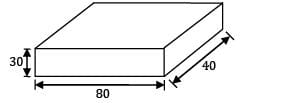Detailed Solution for Test: Casting Level - 3 - Question 1 Since given machining allowance is 3 mm on each side, add 3 mm on each side of the part shown in figure. The dimensions of pattern after machining will be

L = 80 + 2 x 3 = 86 mm

W = 40 + 2 x 3 =46 mm

H = 30 + 2 x 3 = 36 mm

The shrinkage allowance of 3% is added to all the dimensions of pattern. Dimension of pattern after providing shrinkage allowance of 3% will be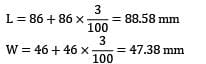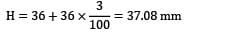Given shaking allowance is 1 mm on length and width. Recall that shaking allowance is a negative allowance. Hence 1 mm has to be reduced from the calculated values of length and width side.

L = 88.58 - 1 = 87.58 mm

W = 47.38 - 1 = 46.38 mm

H = 37.08 mm

*Answer can only contain numeric values
Test: Casting Level - 3 - Question 2

### The ratio of solidification times of a sphere and cube made of same materials cast under identical conditions 2:1, are , the ratio of their volumes are __________

Detailed Solution for Test: Casting Level - 3 - Question 2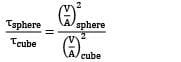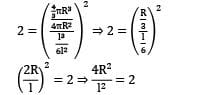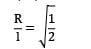R = 0.7071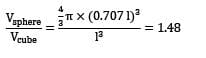*Answer can only contain numeric values
Test: Casting Level - 3 - Question 3

### Metal is to be poured in a cubical cavity of 100 cm side made entirely in drag. The crosssection of the cope box is 200x 150 cm and height is 100 cm. If the densities of metal and sand are 7900 kg/m3 and 1600 kg/m3 respectively. The minimum weight required to be placed on cope in kN to prevent lifting of cope box is __________.

Detailed Solution for Test: Casting Level - 3 - Question 3 Metallastatic pressure on the mould = pgh

= 7900 x 9.81 x 1

= 77499 N/m2

Upward force exerted by molten metal on cope = 77499 x 1 x 1

= 77.499 kN

So, minimum weight to keep in cope box

= 77.499 - 4.8

= 72.699 kN

Test: Casting Level - 3 - Question 4

For a given volume, the ratio of height to diameter of a blind cylindrical riser with a hemispherical top for minimum cooling rate is____________.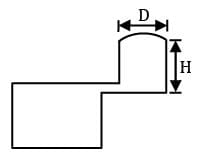Detailed Solution for Test: Casting Level - 3 - Question 4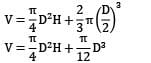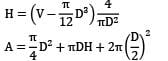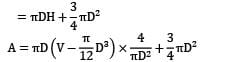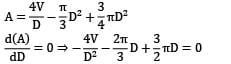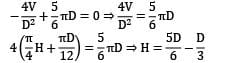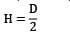*Answer can only contain numeric values
Test: Casting Level - 3 - Question 5

In sand casting of hollow part of zinc, a cylindrical core of diameter 130 mm and height 200 mm is placed inside the mould cavity. The densities of core material and zinc are 1500 kg/m3 and 12000 kg/m3 respectively. The net force that tends to lift the core during pouring of molten metal will be __________ N.

Detailed Solution for Test: Casting Level - 3 - Question 5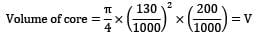Upward force buoyancy force weight of molten metal displaced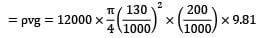Downward force weight of core

D = density of core

= dvg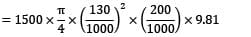Net force upward force – downward force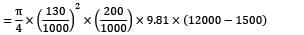= 273.441 N

*Answer can only contain numeric values
Test: Casting Level - 3 - Question 6

A disk of diameter 40 cm and 5 cm thick is to be casted out of pure aluminium is an open mold operation. Melting temperature of Al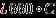, pouring temperature 800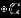. Assume that the amount of aluminium heated will be 5% more than that needed to fill the mould cavity. The amount of heat (MJ) added to aluminium starting from room temperature of 25is__________. (Specific heat of Al (both cold and heated)= 0.88 J/g. Heat of fusion= 389 J/g. Density Al = 2.70 g/cm3 ).

Detailed Solution for Test: Casting Level - 3 - Question 6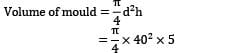Volume of molten metal = 5% more than volume of mould

⇒ Volume of molten metal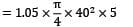Mass of molten metal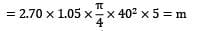heat supplied = m(cp(Tm - TR) +L.H + Cp(TP -Tm))

Tm = melting temperature

TR = room temperature

Tp = pouring temperature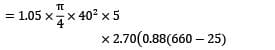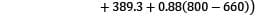= 19 MJ

Test: Casting Level - 3 - Question 7

Two castings of the same metal have the same surface area. One casting is in form of a sphere and the other is a cube. The ratio of the solidification time for the sphere to that of the cube is____________.

Detailed Solution for Test: Casting Level - 3 - Question 7

Let τ = Solidification time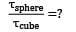According to Chvorinov’s rule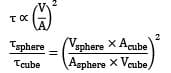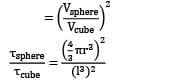But since Acube = Asphere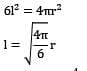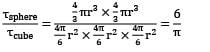Test: Casting Level - 3 - Question 8

Match List-I with List-II and select the correct answer using the codes given below the lists: List-I

P. Shrinkage cavity

Q. Drop

R. Blister

S. Dross

List-II

1. This is a scar covered by thin layers of a metal

2. Lighter impurities appearing on the top surface of a casting

3. An irregularly shaped projection on the cope surface of the casting

4. An improper riser may give rise to this defect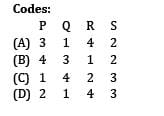Detailed Solution for Test: Casting Level - 3 - Question 8

Shrinkage cavity ➝ if shrinkage of metal is not compensated by riser this defect is caused.

Drop ➝ is an irregularly shaped projection on the cope surface of casting.

Blister ➝ A scar covered by thin layer of metal.

Dross ➝ lighter impurities appearing on the top surface of a casting.

Test: Casting Level - 3 - Question 9

A cylinder of 150 mm diameter & 200 mm height is to be cast without any riser. The cylinder is moulded entirely in drag of a green sand flask & is top gated. The cope of flask is 200 mm height & the height of metal pouring is 50 mm above the cope. A tapered sprue is employed & the gating ratio is 1 : 1.5 : 2. The time taken _________ (in seconds) to fill the casting cavity neglecting energy losses, if the ingate area is 400 sq-mm.

Detailed Solution for Test: Casting Level - 3 - Question 9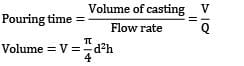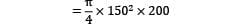Flow rate = Q = Velocity x area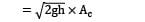Ac = minimum cross sectional area

Gating ratio = 1: 1.5: 2

In gate area = 400 mm2 ⇒ minimum area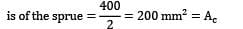Pouring time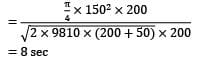*Answer can only contain numeric values
Test: Casting Level - 3 - Question 10

A cube and sphere made of same material cast under identical conditions solidify at the same time. The ratio of length of cube to diameter of sphere is __________

Detailed Solution for Test: Casting Level - 3 - Question 10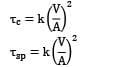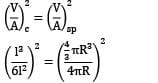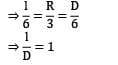*Answer can only contain numeric values
Test: Casting Level - 3 - Question 11

In pressurized gating system with a gating ratio 2:1.5:1. The sprue area is 200mm2 . The time taken (in sec) to fill the cavity of volume 106mm3 with a 20% metal head of 200 mm assuming a 20% loss of head in gating system is __________

Detailed Solution for Test: Casting Level - 3 - Question 11

Given Gating Ratio = 2 : 1.5 : 1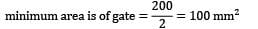Head of metal = 200 mm

loss of head of metal = 20%

net available head = 0.8 x 200

= 160mm

Flow rate of metal = V x A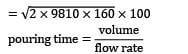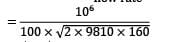= 5.645 sec

*Answer can only contain numeric values
Test: Casting Level - 3 - Question 12

The size of a cylindrical side riser, whose height and diameter are equal, to feed a steel slab casting 30cm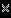30cm6cm is ____________(Use modulus method)

Detailed Solution for Test: Casting Level - 3 - Question 12

The dimensions of the slab

= 30 cm x 30 cm x 6 cm

This can be considered as a long bar with a cross-section of (30 x 6)cm2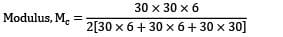= 2.14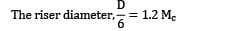D = 6 x 1.2 x 2.14 = 15.408 cm = H

The riser height, H = D = 15.408 cm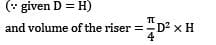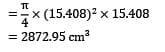*Answer can only contain numeric values
Test: Casting Level - 3 - Question 13

The diameter at base of a down sprue, avoiding aspiration, as shown in figure, to deliver liquid cast iron ( pm=7800 kg/m3 ) at a rate of 10 kg/s against no head at the base of the sprue (Neglecting the friction and orifice effects) is __________.(answer should be in cm)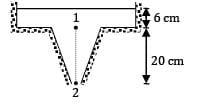Detailed Solution for Test: Casting Level - 3 - Question 13

In order to avoid aspiration effect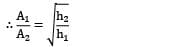Where A1 and A2 are cross-sectional areas at point 1 and 2.

H2 = 20 + 6 = 26 cm

h1 = 6 cm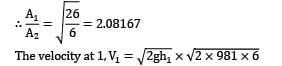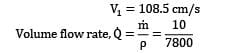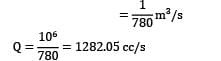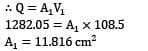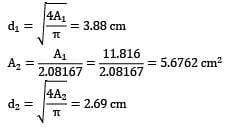*Answer can only contain numeric values
Test: Casting Level - 3 - Question 14

A cylindrical riser is attached to the side of a steel plate casting having dimensions 25cm x 12.5cm x 5cm. The volume shrinkage of steel during solidification is 3% and the volume of riser is 3 times that of dictated by the shrinkage consideration alone. The dimension of the riser in optimum conditions is ____________.

Detailed Solution for Test: Casting Level - 3 - Question 14

For optimum cylindrical side riser,h = d

Volume of casting, Vc = 25 x 12.5 x 5

Shrinkage of steel during solidification = 3%

Minimum volume of the riser necessary is,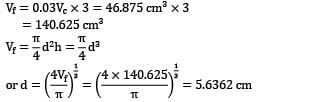*Answer can only contain numeric values
Test: Casting Level - 3 - Question 15

A casting of dimension 200 x 100 x 60mm is casted in such a way that 60 mm dimension is vertical and 2/3 rd of it is in drag. If the height of cope is 100 mm and cavity is fed by a bottom gate with a cross-sectional area 100mm2 , the time (seconds) taken to fill the cavity is _____________.

Detailed Solution for Test: Casting Level - 3 - Question 15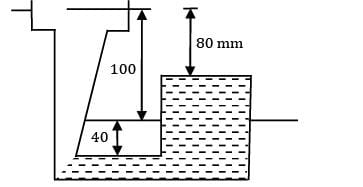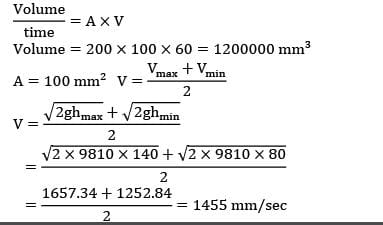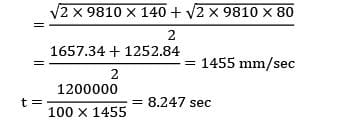Test: Casting Level - 3 - Question 16

Match the following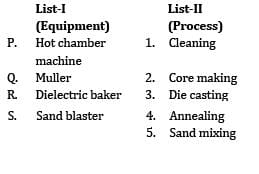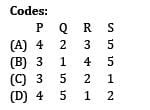Detailed Solution for Test: Casting Level - 3 - Question 16
• Hot chamber machine used for die casting

• Muller is used for mixing sand

• Die electric baker is used for making cores with bentonite as bonding material

• Sand blaster is used for cleaning.

*Answer can only contain numeric values
Test: Casting Level - 3 - Question 17

A casting of size 100cm x 100cm x 25cm was filled by top and bottom gates with manometric height in pouring basin to be 25 cm. The area of gate is 5cm2 . The difference in time (in s) to fill the casting by top and bottom gates is___________.

Detailed Solution for Test: Casting Level - 3 - Question 17

Casting area, A = 100 x 100 = 104cm2

casting height, H = 25 cm

Manometric height, ht = 25 cm

Gate area, Ag = 5 cm2

Top Gate: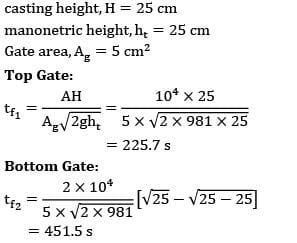Bottom Gate:*Answer can only contain numeric values
Test: Casting Level - 3 - Question 18

The pouring basin in a sand mould is 200 mm diameter & 100 mm height. The mould cavity is a cube of 125 mm side and must be filled in 25 second. The maximum velocity must ensure laminar flow into the mould cavity and in the gates of circular cross section.

Assume:

(A) Constant temperature,

(B) No loss in the velocity head and

(C) Diameter of gate 8 times diameter of the sprue base. Properties of the molten metal are v=0.9mm2 per second,p= 700kg/m3 and cp=33.6 J/mol-k.

The diameter of sprue to avoid aspiration effect is ____________.(fill the values of the 2 integer in between which the answer lies)

Detailed Solution for Test: Casting Level - 3 - Question 18

The dimension of pouring basin will not affect the pouring time

Let V = maximum velocity of molten metal in the gating system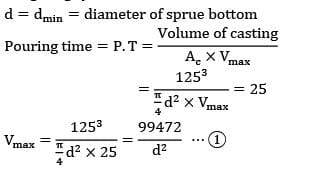To ensure the laminar flow in the gating system Re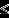2000

Worst case, Re = 2000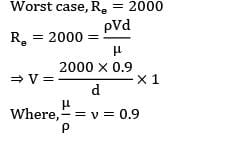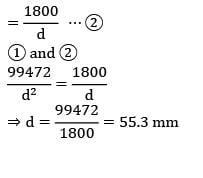Test: Casting Level - 3 - Question 19

Sprue in casting refers to.......

Test: Casting Level - 3 - Question 20

A cylindrical job with diameter of 200 mm and height of 100 mm is to be cast using modulus method of riser design. Assume that the bottom surface of cylindrical riser does not contribute as cooling surface. If the diameter of the riser is equal to its height, then the height of the riser (in mm) is

## Manufacturing Engineering

41 videos|38 docs|27 tests
Information about Test: Casting Level - 3 Page
In this test you can find the Exam questions for Test: Casting Level - 3 solved & explained in the simplest way possible. Besides giving Questions and answers for Test: Casting Level - 3, EduRev gives you an ample number of Online tests for practice

## Manufacturing Engineering

41 videos|38 docs|27 tests(Scan QR code)